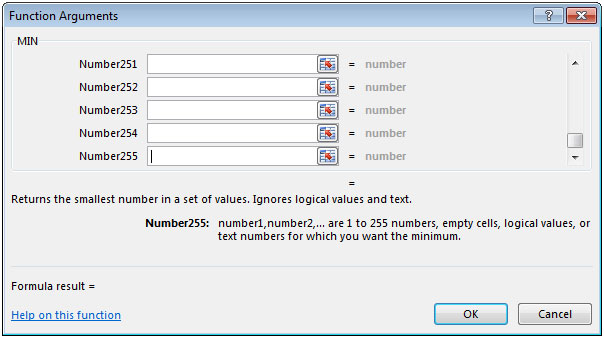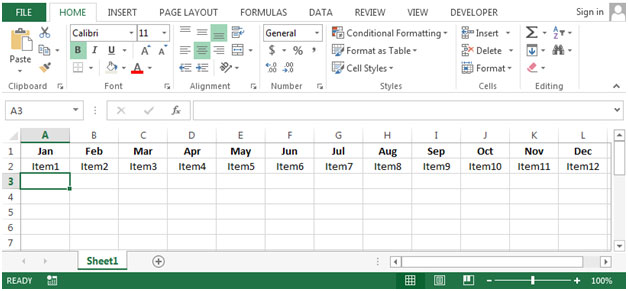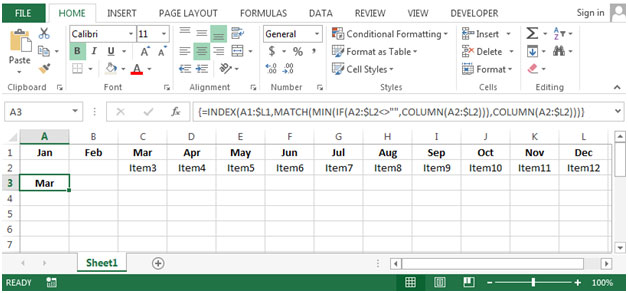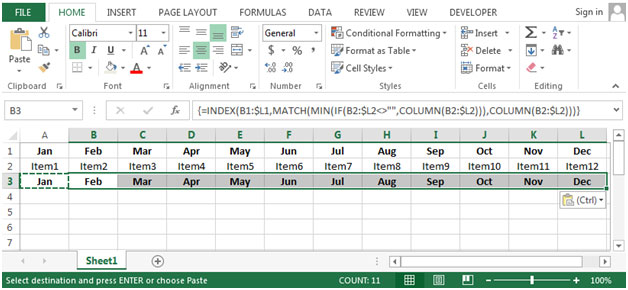# Retrieving the Column Header of the Next Non-Blank Cell in a Row in Excel 2010

To retrieve the column header of the next non blank cell in a row, we can use a combination of INDEX, MATCH, MIN, IF & COLUMN functions to get the output.
INDEX: Returns a value or reference of the cell at the intersection of a particular row and column, in a given range.

Syntax: =INDEX(array,row_num,column_num)
MATCH function searches for a specified item in a selected range of cells, and then returns the relative position of that item in the range.

Syntax =MATCH(lookup_value,lookup_array,match_type)
Min: Returns the smallest number from a range of cells or array. For example,if a list of numbers contains 5, 6, 7, 8, 9 & 10, then the output will be 5.

Syntax =MIN(number1,number2,...)

There can be a maximum 255 arguments.Refer below shown screenshot:The IF function checks if a condition you specify is TRUE or FALSE. If the condition returns TRUE then it returns preset value, and if the condition returns FALSE then it returns another preset value.

Syntax = IF(logical_test,value_if_true,value_if_false)

COLUMN: Returns the column number of a reference.

Syntax: =COLUMN(reference)
Let us take an example:

Row 1 contains list of Months from January to December. Row 2 lists amounts payable over a twelve month period.A blank cell in the row indicates that no payment is due that month.We want to create a new row that, for each month, will display the name of the month in which the next payment is due.• In cell A3 the formula would be
• =INDEX(A1:\$L1,MATCH(MIN(IF(A2:\$L2<>"",COLUMN(A2:\$L2))),COLUMN(A2:\$L2)))
• Press enter on your keyboard.
• The function will return Jan, it means the payment is due for Jan month.• If we remove the item1 & item2 from row 2, then the function will return the Mar.• By copying the formula from cell A3 to range B3:L3, we can return the month for which payment is due.## Users are saying about us...

1.Hi There,

Really very very helpful blog. Kindly share more of such tricks so that we can use excel as a basic powerful tool for day to day work.

Really appreciate your time and efforts!

Cheers!!

SP

2.hi,

i m using the given formula in excel 2013, but its not working.
even i have done copy paste too,